# Central simple algebra

(diff) ← Older revision | Latest revision (diff) | Newer revision → (diff)
A simple associative algebra with a unit element that is a central algebra. Every finite-dimensional central simple algebraover a fieldis isomorphic to a matrix algebra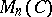over a finite-dimensional central division algebra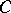over. In particular, ifis algebraically closed, then every finite-dimensional central simple algebraoveris isomorphic to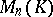, and if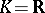, thenis isomorphic to the algebra of real or quaternion matrices. The tensor product of a central simple algebraand an arbitrary simple algebrais a simple algebra, which is central ifis central. Two finite-dimensional central simple algebrasandoverare called equivalent if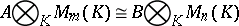for certainand, or, which is equivalent, ifandare isomorphic matrix algebras over one and the same central division algebra. The equivalence classes of central simple algebras overform the Brauer group ofrelative to the operation induced by tensor multiplication.